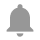Calculator Toolkit
No ratings yet
Version 1.0.0.2Description
`Calculator ToolkitCalculator Toolkit Library includes four VIs: Standard calculator, Scientific calculator, Programmer calculator and Calculate Formula.Standard calculator, Scientific calculator and Programmer calculator can be used to perform calculations using custom formulas.Calculators has four versions of the front panel (user interface), which can be selected by Polymorphic VI selector. Available front panel style options are Custom, Modern, Silver and System.Programmer Calculator can be used to perform boolean calculations using custom formulas.Calculate Formula VI is intended to be used as a subVI for calculations of custom formulas. Calculate Formula VI interprets a string as a formula with variables and calculates the result. Calculator accepts vector variables and performs calculations on arrays. Note, VI Package Manager 2014 is required to install the package.`
Release Notes
```
```